472kHz.org

### Antennas

At least for transmitting a dedicated (tuned) antenna is required.
Due to the wavelength of 630 meter a quarter wave or even a half wave antenna is almost out of order. So most antenna's used on the 472 kHz band are short (compared to the wavelength), resulting in a very small radiation resistance and a large reactive component in the antenna impedance.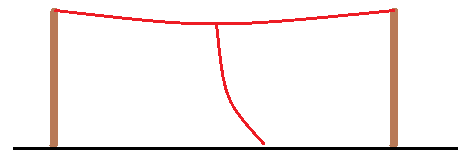The most popular antenna on 630 m is a toploaded vertical monopole. This may sound complicated, but it is a rather simple antenna. In fact it is just a fancy name for one or more horizontal wires (the topload) with somewhere a vertical dropdown wire attached. It can even be as simple as a 80 m or 160 m dipole where the feeding line acts as dropdown wire (just connect both sides of the feeding line together at the lower end).
The basic rules for an efficient 630 m "wire antenna" are:

• Get as much wire as possible as high as possible up in the air.
• Keep away from buildings, trees etc.A short vertical monopole will act as a capacitance (CV) in series with the radiation resistance (RA) and the loss resistance (RG). The antenna current (I) will decrease linear from the feeding point to the top of the antenna, where it will reach 0, while the voltage over the entire antenna will be the same.
The impedance of a short vertical monopole antenna has a capacitive component that is approximately 6 pF per metre. This capacitive component needs to be cancelled out with a series inductance, the so called loading coil (L). The losses in the loading coil will also contribute in the total loss resistance.
On 630 m a good approximation of the radiation resistance of an unobstructed short vertical monopole with no toploading is:

RA = H2/1000
(RA = radiation resistance in Ω, H = antenna height in m)

Be aware that the antenna voltage can be several kV! A 10 m high vertical monopole will have an antenna capacitance of about 60 pF. This will require a 1.87 mH loading coil. The reactance of the loading coil is 5584 Ω (at 475 kHz). If the loss resistance is 40 Ω and the TX power is 100 W the antenna current will be 1.58 A. This current is going through the loading coil and will built up an antenna voltage of 8.82 kV.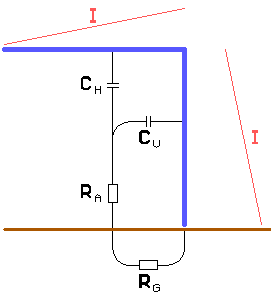The radiation resistance of a short vertical monopole can be improved by increasing the average current. This is done by "toploading" the antenna: adding a horizontal section at the top of the vertical part.

• The loading coil can be smaller, and thus has less loss.
• The antenna voltage will be lower.

The topload will increase the antenna capacitance by about 5 pF per metre.
On 630 m the radiation resistance of a toploaded vertical monopole is approximately:

RA = ((10·LH+6·LV)/(5·LH+6·LV))2·H2/1000
(RA = radiation resistance in Ω, LH = length of the horizontal wire(s), LV = length of the vertical wire, H = antenna height in m)

The loss resistance, that we want to keep as low as possible is determined by:

• The ground conductivity: The better the ground conductivity the lower the losses. Ground conductivity varies from 1000 mS/m (salt water) to 0.01 mS/m (very dry soil).
• Large objects such as buildings and trees: Keeping away from such objects will keep the loss resistance as low as possible.
• The loading coil loss: Using sufficient wire diameter and a good winding technique will keep this loss low. Also the smaller the inductance needed to bring the antenna o resonance the less the loss will be.

In most cases the loss resistance will be in the range of 10 to 100 Ω.

A top loaded vertical monopole can be constructed in many different ways, depending on local conditions. Some varieties: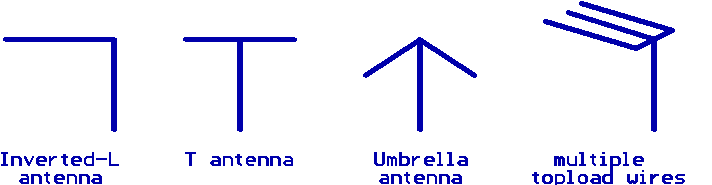A short vertical monopole over a perfect (conducting) ground has a gain of 3 (4.77 dBi, 2.62 dBd) and a take off angle of 0°. As ground conductivity drops the gain will decrease and the take off angle will rise.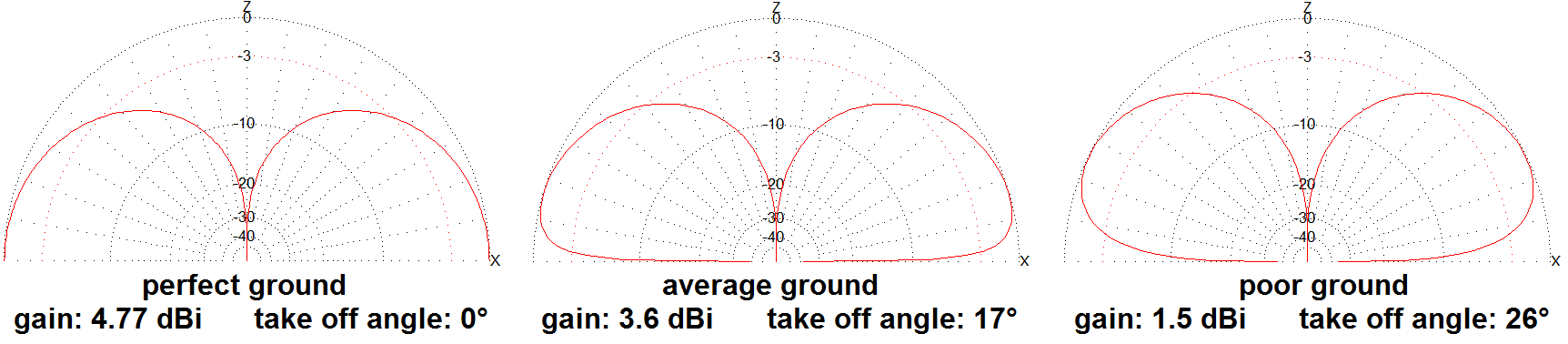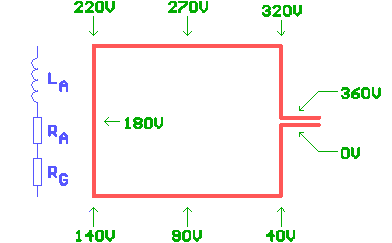An alternative for a (toploaded) vertical monopole is a loop antenna. Here also the rule "the bigger the better" is applicable.
If the loop antenna is small compared to the wavelength (what is very likely on 630 m) the impedance will have an inductive component (LA) that has to be cancelled out with a series capacitor. Note that a small loop antenna will much lower total resistance (RA+RG), compared to a vertical monopole. This results in a high antenna current. As this current also flows through the series capacitor, you will need very decent caps.
The antenna current of a small loop antenna is constant while the antenna voltage will drop lineary.
A loop antenna is far less susceptible to environmental losses, compared to a vertical monopole. As a result the environmental losses are often not dominant compared to the wire resistance loss (including skin effect!) and loss in the series capacitors.
The radiation resistance of a small loop antenna is determined by the loop area A. On 630 m a good approximation of the radiation resistance is:

(Rrad = radiation resistance in Ω, A = loop area in m2)

The inductance of a small loop antenna depends on the shape, size and wire diameter. A good approximation is given by IN3ODT's formula:

L = 0.2·P·ln((3340·A)/(d·P))
(L = loop inductance in µH, P = loop perimeter in m, A = loop area in m2, d = wire diameter in mm)

A small loop antenna has a theoretical gain of 1.5 (1.76 dBi, -0.39 dBd).

Matching small antennas require basically 2 steps:

1. Bring the antenna to resonance (cancel out the reactive component in the antenna impedance). For a short vertical monopole this is done with the loading coil, a small loop antenna will require a series capacitor.
As the bandwidth of a these antennas is rather small you will probably need to adjust resonance depending on the transmitting frequency (QSY) or even when the weather changes. For that reason the loading coil or series capacitor, or at least a part of it, will need to be adjustable.
2. Match the impedance of the resonated antenna to the transmitter impedance (typical 50 Ω). Although this can be done by tapping the loading coil or the series capacitor it is often more convenient to use a matching transformer or eventually a LC-network.
As the antenna impedance will mainly be determined by the loss resistance of the antenna system rather than by the radiation resistance, you might also need to adjust the impedance matching depending on seasonal changes or even weather changes.

An important parameter of a 630 m transmitting antenna is the efficiency. This efficiency (η) is the ratio of the radiation resistance (RA) and the sum of the radiation resistance and loss resistance (RG):

η = RA / (RA+RG)

• The radiation resistance is mainly determined by the antenna height and the ratio of the horizontal (topload) wires versus the height. Typical values are 0.1 to 1 Ω for a small toploaded vertical monopole and 0.001 to 0.1 Ω for a small loop antenna.
• The loss resistance is determined by environmental losses and the loss in the loading coil for a vertical monopole (typical 10 to 100 Ω). For a small loop antenna the wire resistance and series capacitor losses are often dominant (typical 0.1 to 1 Ω).

This means that the typical efficiency of a 630 m transmitting antenna is in the 0.1% (-30 dB) to 10% (-10 dB) range, unless you have a real big antenna where efficiency can be up to 50% or more.

Useful links: ON7YD: Antennas for 136 kHz (an in-depth primer on small antennas, most of the information is also applicable on 472 kHz)
PA3BCA: 500 kHz antenna system @ pa3bca
N6LF: Some Thoughts on 630 m Verticals part 1 & part 2
VK1SV: 472 kHz small loop experiment
SM6BGP: Dynamic Vertical Antenna for 500 kHz (or how to contruct a large antenna for 500/472 kHz)